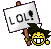Simple AlgebraSimple Algebra

One day, a person went to horse racing area, Instead of counting the number of human and horses, he instead counted 74 heads and 196 legs. Yet he knew the number of humans and horses there. How did he do it, and how many humans and horses are there? IF you cannot solve then send "give up". Once I get a lot of give ups and answers I will give you the answer.shrewdgirl8
Novice PosterPosts : 2
Points : 7
Reputation : 0
Join date : 2009-03-13
Location : uh.. here, duhRe: Simple Algebra

System of equations would be best fit here:

Where x is defined as the number of humans, and y as the number of horses we can derive an equation for the legs and the number of heads:I leave the means of solving the equation to the fantasy of the problem solver (I used elimination):So 50 humans, and 24 horses.

(Just as a side note, because I'm interested in this problem. Name all the ways of solving an equation you can, as in elimination, etc. I believe I may have several newer strategies that are faster for solving larger equations. If you don't know them, that's okay, I'll be posting them if someone is interested. Reply to this topic if interested.)ArchisPosts : 78
Points : 38
Reputation : 4
Join date : 2008-09-29Re: Simple Algebra

that's correctshrewdgirl8
Novice PosterPosts : 2
Points : 7
Reputation : 0
Join date : 2009-03-13
Location : uh.. here, duhRe: Simple Algebra